# 5G NR Downlink ACLR Measurement

This example shows how to measure the adjacent channel leakage ratio (ACLR) for 5G NR test models (NR-TMs) in frequency range 1 (FR1) and FR2 using 5G Toolbox™.

### Introduction

The ACLR is the ratio of the filtered mean power centered on the assigned channel frequency to the filtered mean power centered on an adjacent channel frequency. This example performs ACLR measurements for an NR downlink waveform, as defined in TS 38.104 Section 6.6.3. To model the effect of out-of-band spectral emissions, the example applies spectral regrowth on an oversampled waveform by using a high power amplifier (HPA) model.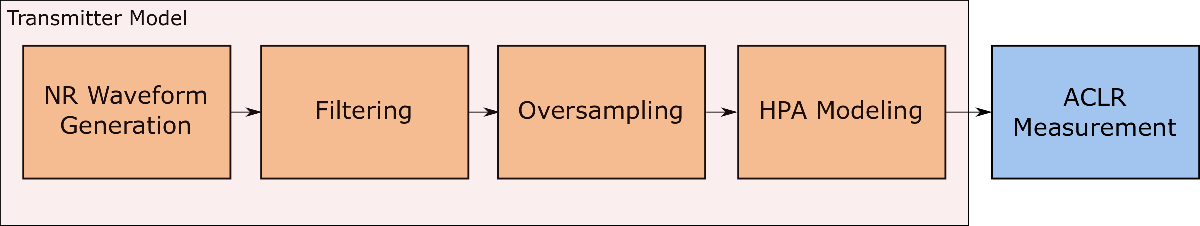### Generate NR-TM Waveform

Use the MATLAB class hNRReferenceWaveformGenerator to generate 5G NR-TMs for FR1 and FR2. You can generate the NR-TM waveforms by specifying these parameters:

• NR-TM name

• Channel bandwidth

• Subcarrier spacing

• Duplexing mode

For more information, see the 5G NR-TM and FRC Waveform Generation example.

```% Select the NR-TM waveform parameters nrtm =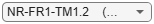"NR-FR1-TM1.2"; % NR-TM name and properties bw =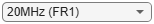"20MHz"; % Channel bandwidth scs =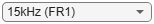"15kHz"; % Subcarrier spacing dm =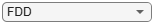"FDD"; % Duplexing mode % Create generator object for the above NR-TM tmWaveGen = hNRReferenceWaveformGenerator(nrtm,bw,scs,dm); % Ensure no windowing to highlight impact of filtering on ACLR tmWaveGen = makeConfigWritable(tmWaveGen); tmWaveGen.Config.WindowingPercent = 0; % Generate waveform [tmWaveform,tmWaveInfo] = generateWaveform(tmWaveGen); samplingRate = tmWaveInfo.Info.SamplingRate; % Waveform sampling rate (Hz) % Visualize the associated PRB and subcarrier resource grids displayResourceGrid(tmWaveGen);```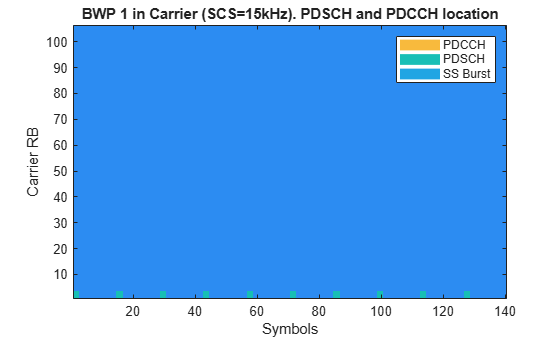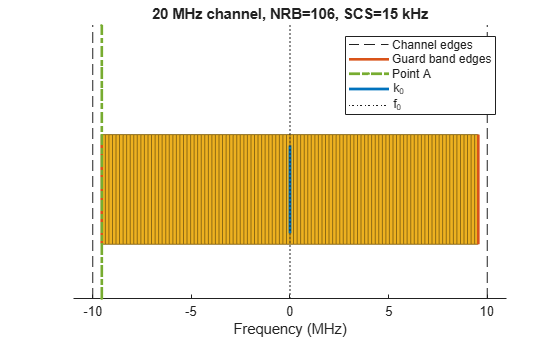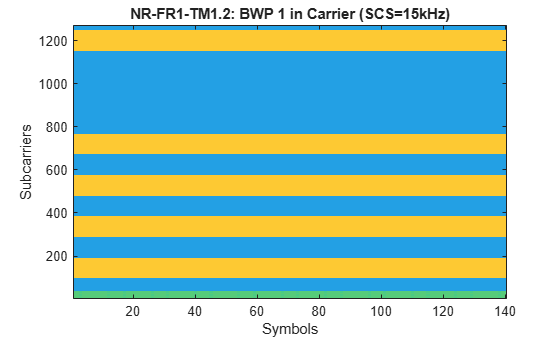#### Display Results

• The associated PRB resource grid (top) depicts the allocation of the different components (PDCCH, PDSCH, CORESET and SS Burst) in each BWP. The grid does not plot the amplitude of the signals only the signal locations in the grid.

• The SCS specific carrier resource grids (middle) along with the minimum guardbands, aligned relative to the overall channel bandwidth.

• The subcarrier resource grid (bottom) indicates the amplitude levels of the generated waveform. If just one color is shown, all the components have the same amplitude.

### Calculate ACLR Parameters

The helper function hACLRParametersNR.m calculates the parameters required for ACLR measurement.

The function determines the required oversampling. If the input waveform sampling rate (`samplingRate`) is not sufficient to span the entire bandwidth (`aclr.BandwidthACLR`) of the adjacent channels (allowing for a maximum of 85% bandwidth occupancy), you can use an upsampled version of the waveform for ACLR calculations. The upsampling factor is `aclr.OSR`.

```aclrParameters = hACLRParametersNR(tmWaveGen.Config); disp(aclrParameters);```
``` Bandwidth: 20000000 SubcarrierSpacing: 15000 BandwidthConfig: 19080000 BandwidthACLR: 100000000 OSR: 4 SamplingRate: 122880000 ```

### Filter Waveform to Improve ACLR

The generated waveform has no filtering, so there are significant out-of-band spectral emissions owing to the implicit rectangular pulse shaping in the OFDM modulation (each OFDM subcarrier has a sinc shape in the frequency domain). Filtering the waveform improves ACLR performance.

Design a filter with a transition band that starts at the edge of the occupied transmission bandwidth (`aclr.BandwidthConfig`) and stops at the edge of the overall channel bandwidth (`aclr.Bandwidth`). This filter involves no rate change, it just shapes the spectrum within the original bandwidth of the waveform.

```% Design filter lpFilt = designfilt('lowpassfir',... 'PassbandFrequency',aclrParameters.BandwidthConfig/2,... 'StopbandFrequency',aclrParameters.Bandwidth/2,... 'PassbandRipple',0.1,... 'StopbandAttenuation',80,... 'SampleRate',samplingRate); % Apply filter filtTmWaveform = filter(lpFilt,tmWaveform);```

### Oversampling and HPA Nonlinearity Model

To create a signal capable of representing 1st and 2nd adjacent carriers, for example, to represent `aclr.BandwidthACLR` with at most 85% bandwidth occupancy, oversample the NR waveform. After oversampling the signal, employ an HPA model to generate out-of-band distortion. For example, to simulate the HPA behaviour, you can use the Rapp method, which is widely used in wireless applications to generate AM/AM distortion. In MATLAB®, you can use the Memoryless Nonlinearity object to model the Rapp method. To highlight the impact of filtering on the ACLR measurements, apply the oversampling and HPA nonlinearities first to the filtered NR signal and then to the same NR signal without filtering.

```% Apply required oversampling resampled = resample(tmWaveform,aclrParameters.OSR,1); % Not filtered filtResampled = resample(filtTmWaveform,aclrParameters.OSR,1); % Filtered % Create and configure a memoryless nonlinearity to model the amplifier nonLinearity = comm.MemorylessNonlinearity; nonLinearity.Method = 'Rapp model'; nonLinearity.Smoothness = 3; % p parameter nonLinearity.LinearGain = 0.5; % dB nonLinearity.OutputSaturationLevel = 2; % It limits the output signal level % Signal conditioning to control the HPA input back-off level resampled = resampled/max(abs(resampled)); % Not filtered filtResampled = filtResampled/max(abs(filtResampled)); % Filtered % Apply the amplifier model to the NR waveforms txWaveform = nonLinearity(resampled); % Not filtered txFiltWaveform = nonLinearity(filtResampled); % Filtered```

### Calculate NR ACLR

The hACLRMeasurementNR.m helper function measures the NR ACLR using a square window on adjacent channels. This function takes a DFT of the measurement signal and uses the energy of the appropriate bins to calculate the adjacent channel powers.

```% Calculate NR ACLR aclr = hACLRMeasurementNR(aclrParameters,txWaveform); % Not filtered filtAclr = hACLRMeasurementNR(aclrParameters,txFiltWaveform); % Filtered```

The `hACLRMeasurementNR.m` helper function returns the ACLR measurements in a structure with these fields:

• `Bandwidth`: the channel bandwidth associated with `tmWaveform`, in Hertz. This is the overall bandwidth of the assigned channel.

• `SubcarrierSpacing`: the subcarrier spacing associated with `tmWaveform`, in Hertz.

• `BandwidthConfig`: the transmission bandwidth configuration associated with `tmWaveform`, in Hertz. This is the bandwidth within the channel bandwidth that contains active subcarriers.

• `BandwidthACLR`: the bandwidth required to represent 1st and 2nd adjacent carriers; the sampling rate used internally for ACLR measurements will support this bandwidth with at most 85% bandwidth occupancy.

• `OSR`: the integer oversampling ratio of the input `tmWaveform` required to create a signal capable of representing 1st and 2nd adjacent carriers.

• `SamplingRate`: the sampling rate of the internal measurement signal from which the ACLR is calculated. If `OSR = 1`, this signal is the input waveform; if `OSR > 1`, this signal is the input waveform upsampled by `OSR`. Therefore: `aclr.SamplingRate = OSR*samplingRate`.

• `CarrierFrequency`: a vector of NR center frequencies, in Hertz, for adjacent channels [-2,-1,1,2].

• `SignalPowerdBm`: the power, in decibels relative to 1 mW in 1 ohm, of the input within the NR channel of interest, for example in a square filter of bandwidth `aclr.BandwidthConfig` centered at 0 Hz.

• `ACLRdB`: a vector of NR ACLRs, in decibels relative to `aclr.SignalPowerdBm`, measured for adjacent channels [-2,-1,1,2].

### Display Results

The hACLRResultsNR.m helper function displays the NR ACLR and plots the NR spectrum and the adjacent channel leakage ratios.

#### Not Filtered

According to TS 38.104 Section 6.6.3.2, the minimum required ACLR for conducted measurements is 45 dB. As some of these ACLR values are lower than 45 dB, they do not fall within the requirements.

`hACLRResultsNR(aclr,txWaveform,'(not Filtered)');`
``` Bandwidth: 20000000 SubcarrierSpacing: 15000 BandwidthConfig: 19080000 BandwidthACLR: 100000000 OSR: 4 SamplingRate: 122880000 CarrierFrequency: [-40000000 -20000000 20000000 40000000] SignalPowerdBm: 19.7642 ACLRdB: [78.1435 41.6526 40.0279 79.6265] ```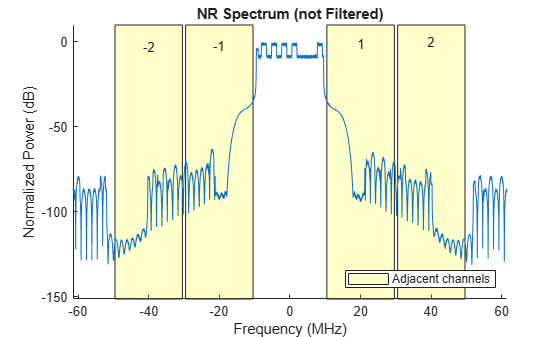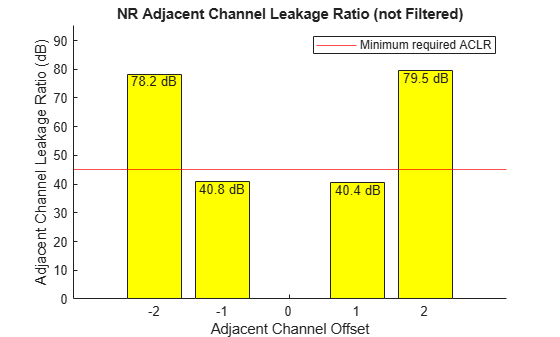#### Filtered

The performance improves when the generated waveform is filtered. The ACLR results with the filtered waveform are higher than the minimum required value.

`hACLRResultsNR(filtAclr,txFiltWaveform,'(Filtered)');`
``` Bandwidth: 20000000 SubcarrierSpacing: 15000 BandwidthConfig: 19080000 BandwidthACLR: 100000000 OSR: 4 SamplingRate: 122880000 CarrierFrequency: [-40000000 -20000000 20000000 40000000] SignalPowerdBm: 19.6869 ACLRdB: [78.1614 71.6984 71.6686 79.6094] ```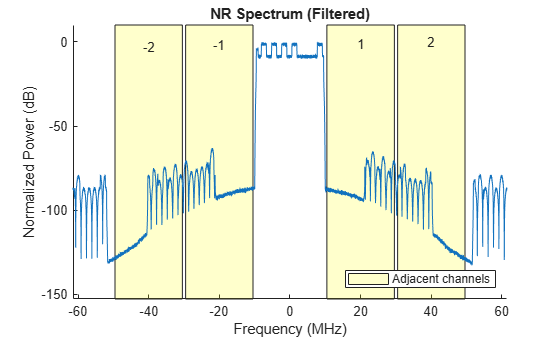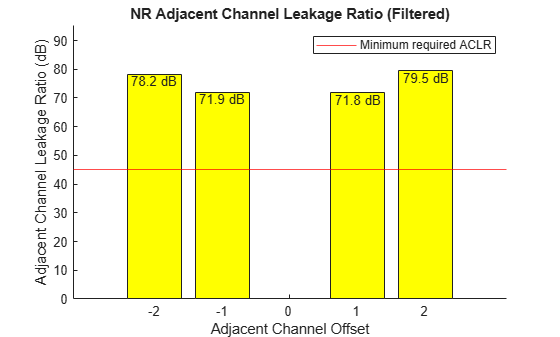### Appendix

This example uses the following helper functions and classes:

• hACLRMeasurementNR.m

• hACLRParametersNR.m

• hACLRResultsNR.m

• hNRReferenceWaveformGenerator.m

 3GPP TS 38.104. “NR; Base Station (BS) radio transmission and reception.” 3rd Generation Partnership Project; Technical Specification Group Radio Access Network.